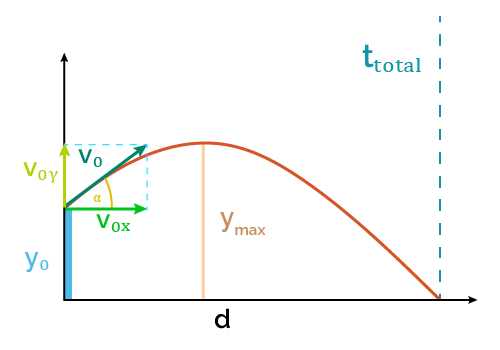# Maximum Height Calculator - Projectile Motion

Created by Luis Hoyos
Last updated: Jul 28, 2022

If you're searching for how to find the maximum height in projectiles, this maximum height calculator is what you need. This tool is a simplified version of our projectile motion calculator, which finds many other parameters using different projectile motion formulas.

Read on to know the maximum height formula this physics calculator uses!

## Projectile motion formulas

To calculate the maximum height, we first must know the fundamental equations behind the projectile motion.

The following table contains the formulas that govern this form of motion:

 Horizontal motion x = x0 + Vxt Vx = V0x Vertical motion y = y0 + (1/2)(V0y + Vy)t Vy = V0y - gt y = y0 + V0yt - gt2/2 Vy2 = V0y2 - 2g(y - y0)

In the previous table:

• t — time;
• x and y — Horizontal and vertical positions, respectively (at the time t);
• x0 and y0Initial horizontal and vertical positions;
• Vx and Vy — Horizontal and vertical velocities, respectively (at the time t);
• V0x and V0yInitial horizontal and vertical velocities, respectively; and
• g — Gravity acceleration (9.807m/s2)

Usually, we don't know the horizontal and vertical velocity components in advance. As those components form right triangles with the initial total velocity (V0), we can calculate them using the following formulas:

• Horizontal velocity formula: V0x = V0 × cos(α)
• Vertical velocity formula: V0y = V0 × sin(α)

where α is the projectile angle of launch.

If the vertical velocity component is zero (α = 0°), then that's a case of horizontal projectile motion, and if α = 90°, that's a case of free fall.

Now, let's see how to find the maximum height.

## Maximum height formula

The formula to calculate the maximum height of a projectile is:

• ymax = y0 + V0y²/(2g); or
• ymax = y0 + V02sin2α/(2g)

where:

• y0Initial height or vertical position;
• V0y — Initial vertical velocity;
• V0 — Initial total velocity (called initial velocity in the maximum height calculator);
• g — Gravity acceleration;
• α — Angle of launch; and
• ymax — Calculated maximum height;

And that's it! That's the maximum height formula for physics problems involving projectile motion.

Luis Hoyos
Velocity (V₀)
ft/s
Angle of launch (α)
deg
Initial height (y₀)
ft
Maximum height
Maximum height (yₘₐₓ)
ftPeople also viewed…

### Conservation of momentum

Learn how to calculate the momentum of an object in a closed system with our conservation of momentum calculator.